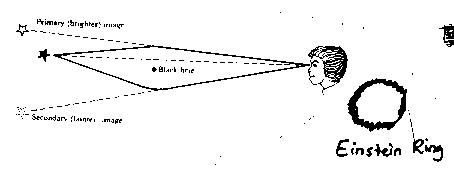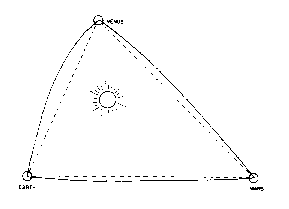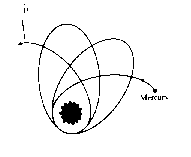Einstein and Modern Theories of Gravity

You are probably familiar with Einstein's famous equation:

E = mc²

where E represents energy, m represents mass, and c represents the speed of light in a vacuum. This equation implies that energy and mass are essentially two forms of the same thing. Since gravity acts on anything with mass, it must also act on anything with energy, since associated with this energy there is an "effective" mass. Light is something that has energy but no actual mass. According to Einstein, however, light must have an "effective" mass, calculated by E = mc². If it has an effective mass, its path of motion must be bent by gravity as it passes by very massive objects.

In fact this is true: the path of light is bent by gravity. One result of this is gravitational lensing, which is the idea that we can see a star that is directly behind a very massive object (like a black hole), because the light from the star may be bent by gravity around the black hole just enough for it to reach our telescopes. Astronomers have observed "the Einstein ring", which is a ring of light coming such a star. We see a ring because the star and the black hole are so perfectly aligned that we get light bent around all "sides" of the black hole towards us.

Diagram #12: Gravitational lensing(from Universe by Kaufmann)

Because light is affected by gravity, another prediction is that as a photon travels away from it's very massive source, it should "slow down" because it is being attracted to it's source. We know that light does not slow down as it loses energy, however: it's speed is a constant in a given medium. However, it may lose energy by shifting to a lower frequency (or a longer wavelength). This is called a gravitational redshift, because in the visible spectrum, the longer wavelength corresponds to a "more red" part of the spectrum. This gravitational redshift has been confirmed experimentally.

Another theory of Einstein's is that space is curved. You can see how putting a curve in 2-D space (as in the case of the gravity well) mimics the effects of gravity. Similarly, putting a curve in 3-D space mimics the effect of gravity in our three dimensional world. This is hard to visualize, but the following explanation may help. We know light is curved by gravity. Imagine one person on Venus, one person on Mars, and one person on Earth, with the Sun inbetween these planets, as shown in the diagram below. If each person measures the angles between the other two planets from their place, we could draw a triangle. However, the triangle will have curved sides, because the path of light from each planet to another is bent by the Sun's gravitational field. We would have a triangle whose angles do not equal 180 degrees! However, we know the lines must be straight, because a straight line is defined as along the line of sight. These must be straight lines, in curved space.

Diagram # 13: The curvature of space.(from The Great Physicists from Galileo to Einstein by George Gamow)

Sound confusing? Try a short experiment with a balloon. Before you blow it up, draw a triangle on it, and make sure all of the lines are straight. If you then inflate the balloon, bending the space, you will end up with a "curved triangle", similar to the one just described. By putting a curve in 2-D space, you end up with "straight" lines in curved space. In fact there is evidence to support this theory as well. Astronomers in this century have very detailed information about the orbits of the planets. It has been known for some time that the ellipse of Mercury's orbit, which is highly elliptical, slowly rotates around it's major axis. If you can find a spirograph set, sold in toy stores, this is a super way to demonstrate this effect.

Diagram #14: Mercury's orbit(from Universe by Kaufmann)

Mercury's orbit rotates around it's axis mainly because of the gravitational interactions with the other planets. Remember, when we consider outer space, there are not just two massive objects to consider: there are many, and they all interact. However, detailed calculations of Mercury's orbits did not quite match the observations: they were off by about 43 arcseconds per century. (There are 60 arcminutes in a degree of angle, and there are 60 arcseconds in an arcminute.) As you can see, the calculations are off by a very tiny amount, but physicists like to worry about details like this. Einstein's Theory of General Relativity was able to explain why the discrepancy was exactly 43 arcseconds.

Einstein also predicted that gravity must affect time, as well as space. He predicted that time passes more slowly in a strong gravitational field, so a clock on the ground floor of a tall building would tick slightly slower than a clock on the top of the building. This effect is very minimal, but experiments have also confirmed this prediction.

Yet another prediction is that by moving an object or changing it's gravitational field, gravity "waves" can be created. Pretend that your hand is incredibly massive, and then think about moving it up and down. As you move it, you are moving the warp in space that your gravitational field has created. This change in the warp must affect the space beside it, and so you will end up with a ripple of this changed warp propagating through space. Although gravitational waves are extremely weak, they have been detected experimentally.

So did Einstein discover everything? Well, not quite. As you saw, his theories explained some very minute discrepancies between observations and previous theories. His theories also introduce an interesting new way of viewing things. However, there are many details still to be explained. Physicists not only try to explain phenomena to great precision, and under all extremes of conditions, but they also try to find how things are related. You will recall that all of the conics are related by one formula, even though their shapes appear to be very different. One of the principle goals of physicists now is to come up with a "Theory of Everything" (TOE), also known as the "Grand Unification Theory", or "Unified Field Theory". The goal of this theory is to relate the four forces: gravity, electromagnetic, and the two nuclear forces (electrostrong and electroweak) into one, unified theory. Einstein worked on this for about his last 40 years, but he never solved this problem. Thousands of physicists are still working on this problem.